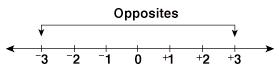# Multiplying Integers

## Learn About Multiplying Integers With The Following Examples And Interactive ExercisesProblem: Alicia owes \$6 to each of 4 friends. How much money does she owe?

Solution: The problem above can be solved using integers.

Owing \$6 can be represented by -6. Thus the problem becomes:

(-6) (+4)

The parentheses indicate that these integers are being multiplied. In order to solve this problem, we need to know the rules for multiplication of integers.

Rule 1: The product of a positive integer and a negative integer is a negative integer.

Rule 2: The product of two negative integers or two positive integers is a positive integer.

We can now use Rule 1 to solve the problem above arithmetically:   (-6) (+4) = -24. So Alicia owes \$24. Let's look at some more examples of multiplying integers using these rules.

Example 1: Find the product of each pair of integers.

 Multiplying Integers Integers Product Rule Used (+7) (+3) = +21 Rule 2 (+7) (-3) = -21 Rule 1 (-7) (+3) = -21 Rule 1 (-7) (-3) = +21 Rule 2

Example 2: Find the product of each pair of integers.

 Multiplying Two Integers Integers Product Rule Used (+8) (+4) = +32 Rule 2 (+11) (-2) = -22 Rule 1 (-14) (+3) = -42 Rule 1 (-9) (-5) = +45 Rule 2

In each of the above examples, we multiplied two integers by applying the rules at the top of the page. We can multiply three integers, two at a time, applying these same rules. Look at the example below.

Example 3: Find the product of each set of integers.

 Multiplying Three Integers Integers Product of First Two Integers and the Third Product (+5) (+3) (+2) = (+15) (+2) = +30 (+8) (+2) (-5) = (+16) (-5) = -80 (-6) (+3) (+4) = (-18) (+4) = -72 (-9) (-3) (+2) = (+27) (+2) = +54 (-4) (-3) (-5) = (+12) (-5) = -60

The Associative Law of Multiplication applies to integers. In Example 3 above, we multiplied the product of the first and second integer by the third integer. We can also solve these problems by multiplying the first integer by the product of the second and third. We will do this in Example 4 below.

Example 4: Find the product of each set of integers.

 Multiplying Three Integers Integers Product of First Integer and the Last Two Product (+5) (+3) (+2) = (+5) (+6) = +30 (+8) (+2) (-5) = (+8) (-10) = -80 (-6) (+3) (+4) = (-6) (+12) = -72 (-9) (-3) (+2) = (-9) (-6) = +54 (-4) (-3) (-5) = (-4) (+15) = -60

Summary: Multiplying two integers with like signs yields a positive product, and multiplying two integers with unlike signs yields a negative product. We can multiply three integers, two at a time, applying these same rules.

### Exercises

Directions: Read each question below. Click once in an ANSWER BOX and type in your answer; then click ENTER. After you click ENTER, a message will appear in the RESULTS BOX to indicate whether your answer is correct or incorrect. To start over, click CLEAR.

 1.(-2) (+7) = ? ANSWER BOX:   RESULTS BOX:
 2.(-8) (-9) = ? ANSWER BOX:   RESULTS BOX:
 3.(+9) (+7) = ? ANSWER BOX:   RESULTS BOX:
 4.(+16) (-3) = ? ANSWER BOX:   RESULTS BOX:
 5.(+4) (-5) (-2) = ? ANSWER BOX:   RESULTS BOX: